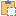#### BoundingBoxXYZ Class

A three-dimensional rectangular box.

Namespace: Autodesk.Revit.DB
Assembly: RevitAPI (in RevitAPI.dll) Version: 2015.0.0.0 (2015.0.0.0)

# Syntax

C#
``public class BoundingBoxXYZ : APIObject``
Visual Basic
``````Public Class BoundingBoxXYZ _
Inherits APIObject``````
Visual C++
``public ref class BoundingBoxXYZ : public APIObject``

# Remarks

The box is defined as follows. A Transform specifies the coordinate system in which the box is defined. The minimum and maximum values for each coordinate in that system are specified. An alternative way to construct the box is: for each basis vector of the transform, there are two planes that are orthogonal to the vector and have their origins given by Transform.Origin + BasisVector * Bound. The entire box and each face may be turned on or off.

# ExamplesCopy C#
``````Document doc = uiApplication.ActiveUIDocument.Document;
View3D view3D = null;
FilteredElementCollector collector = new FilteredElementCollector(doc);
ICollection<Element> collection = collector.OfClass(typeof(View3D)).ToElements();
foreach (Element element in collection)
{
view3D = element as View3D;
if (null != view3D)
{
string messageInfo = "BoundingBoxXYZ : ";
messageInfo += "\nView name : " + view3D.Name;
BoundingBoxXYZ boundingBox = view3D.GetSectionBox();
if (!boundingBox.Enabled)
{
boundingBox.Enabled = true;
}
if (boundingBox.Enabled)
{
// Get max boundingbox
XYZ max = boundingBox.Max;
messageInfo += "\nMax boundingbox : (" + max.X + ","
+ max.Y + "," + max.Z + ")";
// Get min boundingbox
XYZ min = boundingBox.Min;
messageInfo += "\nMin boundingbox : (" + min.X + ","
+ min.Y + "," + min.Z + ")";
// Get if max boundingbox enabled,
// use 0 for X, 1 for Y and 2 for Z
bool bMaxEnabledX = boundingBox.get_MaxEnabled(0);
// Get if min boundingbox enabled,
// use 0 for X, 1 for Y and 2 for Z
bool bMinEnabledX = boundingBox.get_MinEnabled(0);
// Get if bound boundingbox enabled
// For bound, use 0 for Min and 1 for Max.
// For dimension, use 0 for X, 1 for Y and 2 for Z
bool bBoundEnabled = boundingBox.get_BoundEnabled(0, 0);
// Get minimum bound of boundingbox
XYZ minBound = boundingBox.get_Bounds(0);
// Get maximum bound of boundingbox
XYZ maxBound = boundingBox.get_Bounds(1);
}
}
}``````Copy VB.NET
``````Dim doc As Document = uiApplication.ActiveUIDocument.Document
Dim view3D As View3D = Nothing
Dim collector As New FilteredElementCollector(doc)
Dim collection As ICollection(Of Element) = collector.OfClass(GetType(View3D)).ToElements()
For Each element As Element In collection
view3D = TryCast(element, View3D)
If view3D IsNot Nothing Then
Dim messageInfo As String = "BoundingBoxXYZ : "
messageInfo += vbLf & "View name : " + view3D.Name
Dim boundingBox As BoundingBoxXYZ = view3D.GetSectionBox()
If Not boundingBox.Enabled Then
boundingBox.Enabled = True
End If
If boundingBox.Enabled Then
' Get max boundingbox
Dim max As XYZ = boundingBox.Max
messageInfo += ((vbLf & "Max boundingbox : (" + max.X & ",") + max.Y & ",") + max.Z & ")"
' Get min boundingbox
Dim min As XYZ = boundingBox.Min
messageInfo += ((vbLf & "Min boundingbox : (" + min.X & ",") + min.Y & ",") + min.Z & ")"
' Get if max boundingbox enabled,
' use 0 for X, 1 for Y and 2 for Z
Dim bMaxEnabledX As Boolean = boundingBox.MaxEnabled(0)
' Get if min boundingbox enabled,
' use 0 for X, 1 for Y and 2 for Z
Dim bMinEnabledX As Boolean = boundingBox.MinEnabled(0)
' Get if bound boundingbox enabled
' For bound, use 0 for Min and 1 for Max.
' For dimension, use 0 for X, 1 for Y and 2 for Z
Dim bBoundEnabled As Boolean = boundingBox.BoundEnabled(0, 0)
' Get minimum bound of boundingbox
Dim minBound As XYZ = boundingBox.Bounds(0)
' Get maximum bound of boundingbox
Dim maxBound As XYZ = boundingBox.Bounds(1)
End If